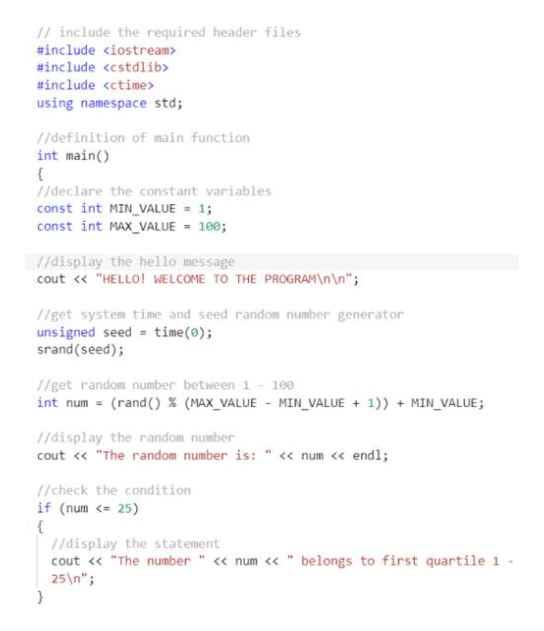# Write a program in c++ to generate a random number between 1 - 100, and then display which quartile the number falls in.First quartile is 1 - 25Second quartile is 26 - 50Third quartile is 51 - 75Fourth quartile is 76 - 100The following program shows how to get a random integer between 1 - 100. Use the code as an example for your own program.SAMPLE PROGRAM// This program displays a random number between 1 - 100#include <iostream> // for input and output#include <cstdlib>  // for rand() and srand()#include <ctime>    // for time()using namespace std;int main(){    //constantsconst int MIN_VALUE = 1;const int MAX_VALUE = 100;//get system time and seed random number generatorunsigned seed = time(0);srand(seed);//get random number between 1 - 100int num = (rand() % (MAX_VALUE - MIN_VALUE + 1)) + MIN_VALUE;cout << num << endl;return 0;}Your program should:contain header comments as shown in classdisplay a "hello" messagegenerate a random number between 1 - 100display the number back to the userdetermine which quartile the number falls in (using any combination of if, else, and else if)display the quartile back to the userdisplay a "goodbye" message before exiting the program

Question

Write a program in c++ to generate a random number between 1 - 100, and then display which quartile the number falls in.

• First quartile is 1 - 25
• Second quartile is 26 - 50
• Third quartile is 51 - 75
• Fourth quartile is 76 - 100

The following program shows how to get a random integer between 1 - 100. Use the code as an example for your own program.

SAMPLE PROGRAM
// This program displays a random number between 1 - 100
#include <iostream> // for input and output
#include <cstdlib>  // for rand() and srand()
#include <ctime>    // for time()
using namespace std;

int main()
{
//constants
const int MIN_VALUE = 1;
const int MAX_VALUE = 100;

//get system time and seed random number generator
unsigned seed = time(0);
srand(seed);

//get random number between 1 - 100
int num = (rand() % (MAX_VALUE - MIN_VALUE + 1)) + MIN_VALUE;
cout << num << endl;

return 0;
}

• display a "hello" message
• generate a random number between 1 - 100
• display the number back to the user
• determine which quartile the number falls in (using any combination of if, else, and else if)
• display the quartile back to the user
• display a "goodbye" message before exiting the program

check_circle

Step 1

Program plan:

• Include the required header files into the program.
• Define the main function.
• Declare the required constant variables
• Display the hello message to the user.
• Get the system time and seed random number generator.
• Get the random number between 1 to 100.
• Display the random number to the user.
• Check the “num” value is less than or equal to 25.
• Display the “num” is belongs to first quartile.
• Check the “num” value is greater than 25 and less than or equal to 50.
• Display the “num” is belongs to second quartile.
• Check the “num” value is greater than 50 and less than or equal to 75.
• Display the “num” is belongs to third quartile.
• Check the “num” value is greater than 75 and less than or equal to 100.
• Display the “num” is belongs to fourth quartile.
• Finally display the goodbye message to the user
Step 2

Program:

// include the required header files

#include <iostream>

#include <cstdlib>

#include <ctime>

using namespace std;

//definition of main function

int main()

{

//declare the constant variables

const int MIN_VALUE = 1;

const int MAX_VALUE = 100;

//display the hello message

cout << "HELLO! WELCOME TO THE PROGRAM\n\n";

//get system time and seed random number generator

unsigned seed = time(0);

srand(seed);

//get random number between 1 - 100

int num = (rand() % (MAX_VALUE - MIN_VALUE + 1)) + MIN_VALUE;

//display the random number

cout << "The random number is: " << num << endl;

//check the condition

if (num <= 25)

{

//display the statement

cout << "The number " << num << " belongs to first quartile 1 - 25\n";

}

//check the condition

else if (num > 25 && num <= 50)

{

//display the statement

cout << "The number " << num << " belongs to second quartile 26 - 50\n";

}

//check the condition

else if (num > 50 && num <= 75)

{

//display the statement

cout << "The number " << num << " belongs to third quartile 51 - 75\n";

}

//check the condition

else if (num > 75 && num <= 100)

{

//display the statement

cout << "The number " << num << " belongs to fourth quartile 76 - 100\n";

}

//display the goodbye message

cout << "\nGOODBYE!";

//return statement

return 0;

}

Step 3

Program Screenshot #1:...help_outlineImage Transcriptionclose/ include the required header files #include #include #include using namespace std 1/definition of main function int main { 1/declare the constant variables const int MIN VALUE 1; const int MAX_VALUE 100; /display the hello message cout << "HELLO! WELCOME TO THE PROGRAM\n\n"; get system time and seed random number generator unsigned seed time(o); srand(seed); /get random number between 1 180 int num (rand) (MAX_VALUE - MIN_VALUE + 1)) MIN VALUE; /display the random number "The random number is: "

### Want to see the full answer?

See Solution

#### Want to see this answer and more?

Solutions are written by subject experts who are available 24/7. Questions are typically answered within 1 hour.*

See Solution
*Response times may vary by subject and question.
Tagged in

### Computer Science